## 4.7.1 Carrier Density

The GREEN's function matrices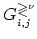are defined in the basis set of ring numbers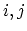and subbands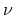. Thus the diagonal elements correspond to the spectrum of carrier occupation (3.79) of those basis sites with a given energy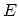. So the total electron and hole density (per unit length) at a site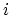is given by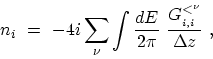(4.50)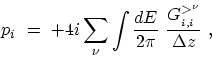(4.51)

where the summation runs over all the subbands contributing to transport and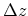is the average distance between rings (4.3). The factor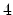in (4.50) and (4.51) is due to double spin and double subband degeneracy (Section 2.4.2). To evaluate these integrals numerically the energy grid should be selected such that the numerical error of the calculation can be controlled. This issue is discussed in Section 4.8. M. Pourfath: Numerical Study of Quantum Transport in Carbon Nanotube-Based Transistors